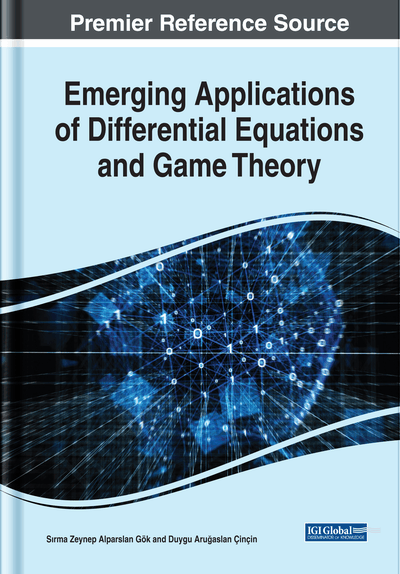# COGARCH Models: An Explicit Solution to the Stochastic Differential Equation for Variance

Yakup Arı (Alanya Alaaddin Keykubat University, Turkey)
DOI: 10.4018/978-1-7998-0134-4.ch005
Available
\$37.50
No Current Special Offers

## Abstract

In this chapter, the features of a continuous time GARCH (COGARCH) process is discussed since the process can be applied as an explicit solution for the stochastic differential equation which is defined for the volatility of unequally spaced time series. COGARCH process driven by a Lévy process is an analogue of discrete time GARCH process and is further generalized to solutions of Lévy driven stochastic differential equations. The Compound Poisson and Variance Gamma processes are defined and used to derive the increments for the COGARCH process. Although there are various parameter estimation methods introduced for COGARCH, this study is focused on two methods which are Pseudo Maximum Likelihood Method and General Methods of Moments. Furthermore, an example is given to illustrate the findings.
Chapter Preview
Top

## Introduction

Mandelbrot (1963) pointed out that the financial time series are not usually stationary, and the increments of these series have no autocorrelation but their squares present out a correlation. Furthermore, he showed the volatility of financial series which exist in volatility cluster is not constant and the distribution of the series is not normal since leptokurtic shape. Black (1976) showed leverage-effect is another stylized fact of financial series. The leverage-effect occurs when the negative fluctuations on stock price have a greater influence on volatility than positive fluctuations do where negative and positive fluctuations have the same magnitude. The long-term persistence is another important subject to discuss for financial series. Fractal mathematics helps us to identify the long-term persistence via models which are the generalization of short-term memory models such as Autoregressive Integrated Moving Average (ARIMA) models with Chaos theory. The randomness generated by deterministic systems, as in how the sensitivity of chaotic systems to their initial conditions can be assumed as a definition of Chaos theory. After the study of Hosking (1981) that is the introduction of the fractional differentiation operator makes these models especially important. The chaotic systems, which have complex forms, are becoming indistinctive with respect to the randomness when the time is going up. Thus, the term pseudo-randomness is sometimes used to define the behaviour of chaotic systems. On the other hand, the scope of non-linear models is the non- stationary of increments assuming the presence of heteroskedasticity in the model. The Autoregressive Conditional Heteroscedastic (ARCH), Generalized ARCH (GARCH) and Continuous GARCH (COGARCH) models and their extensions are the part of this approach.

Engle (1982), The Nobel Prize laureates, has introduced the ARCH models that are proper to represent the typical empirical findings of financial time series. Although the ARCH model can yield volatility clusters, it also has some weaknesses in describing the stylized fact of financial series. It can be concluded that a mechanical way to describe the behaviour of the conditional variance. But it gives no indication of the behaviour of conditional variance because it assumes that positive and negative shocks have the same effects on volatility since it depends on the square of the previous shocks (Tsay, 2013).

After Engle, Bollerslev (1986) whose study was another milestone for financial time series analysis was GARCH model. The discrete-time GARCH models, which are improper models to deal with heteroscedasticity in time series, capture some of the most prominent features in financial data, particularly in the volatility process. In ordinary discrete time GARCH models, time series are assumed to be equally spaced. But time series have often irregular space between two observations. Tick-by-tick data and daily data are examples of this situation. To accommodate the irregularity of time spaces, Klüppelberg et al. (2004) proposed a continuous time GARCH (COGARCH) process driven by a Lévy process, which is an analogue of discrete time GARCH process and is further generalized to solutions of Lévy driven stochastic differential equations. Like in the discrete time case, the COGARCH model cannot model this stylized fact of time series. Therefore, Haug (2006) have developed the exponential COGARCH model as the first extension of the COGARCH model that is based on the discrete time EGARCH model.

The aim of this chapter is to define and to discuss the properties of COGARCH models with examples. In this context, the outline of the chapter is designed as follows;

The general form of the stochastic differential equations (SDEs) is given in Section 2. Then, the properties of the discrete time GARCH model is discussed in section 3. COGARCH process is a Lévy-driven process, therefore the basis of this process is constructed on Compound Poisson and Variance Gamma which are also stochastic processes like Brownian Motion, are defined in Section 4. In the subsection 4.1, derivation of Continuous time GARCH Process and second-order properties of the volatility process will be defined and discussed, depending on the approach of Klüppelberg et al. (2004) and Klüppelberg et al. (2011). Moreover, some classical and Bayesian estimation methods for COGARCH models will be mentioned in Section 5. Compound Poisson COGARCH(1, 1) and Variance Gamma COGARCH(1, 1) simulations will be given as examples and furthermore, the model will be applied to real-life data.

## Complete Chapter List

Search this Book:
Reset